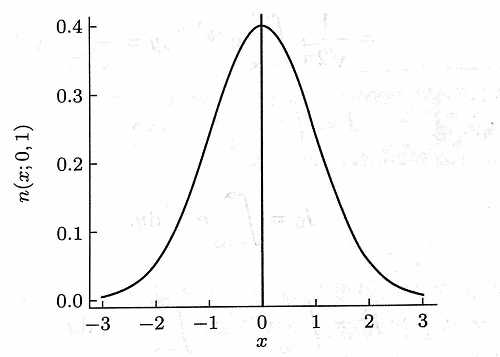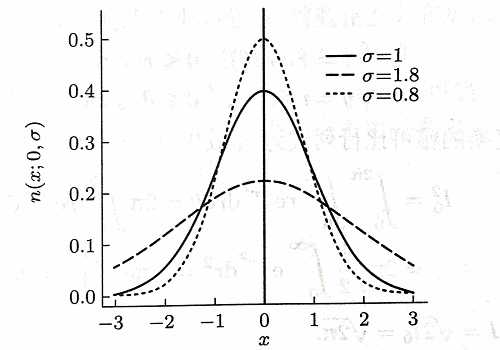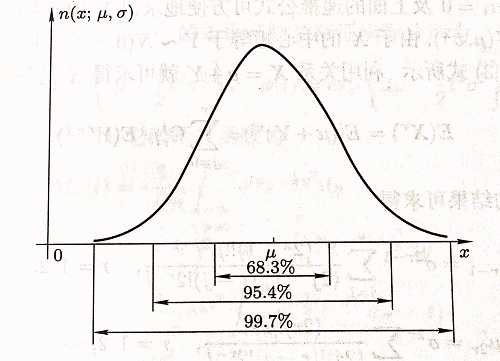## 正态分布的定义

$n(x ; \mu, \sigma)=\frac{1}{\sqrt{2 \pi \sigma}} \exp \left(-\frac{1}{2 \sigma^{2}}(x-\mu)^{2}\right), \quad-\infty

$\exp (x)=e^{x}$

$\mu=0, \sigma=1$ 时， 相应的分布 $N(0, 1)$ 叫做标准正态分布。常用 $Z$ 表示服从标准正态分布的随机变量。

## 正态分布的性质

### 性质1 基本性质

$n (x ; \mu, \sigma)$ 的图形如下图所示：1. 只有一个峰，峰值在 $x = \mu$ 处。这是因为函数 $e^{-x^{2}}$$x=0$ 处达到最大，故 $n (x ; \mu, \sigma)$$x = \mu$ 处达到最大，从而它的众数的 $\mu$
2. 图形关于直线 “$x = \mu$”对称；
3. 图形无论向左或向右延伸，都愈来愈接近横轴，但是不会与横轴相交，即以横轴为渐近线；
4. 参数 $\sigma$ 决定了 $n (x ; \mu, \sigma)$ 图形的形状，$\sigma$ 越大，图形越分散；$\sigma$ 越小，图形越集中，如下图：5. $\varphi(x)$$n (x ; 0, 1)$，则

\begin{aligned} \varphi^{\prime}(x) &=-x \varphi(x) \\ \varphi^{\prime \prime}(x) &=\left(x^{2}-1\right) \varphi(x) \end{aligned}

$\varphi^{\prime \prime}(x) = 0$ 有两个解 $x=\pm 1$，即 $x=\pm 1$ 处有两个拐点。一般地，$n (x ; \mu, \sigma)$$x=\mu \pm \sigma$ 处有两个拐点。

### 性质2 一般正态分布转化标准正态分布

$X \sim N\left(\mu, \sigma^{2}\right)$，则 $Z=(X-\mu) / \sigma \sim N(0,1)$。反之，若 $Z \sim N(0,1)$，则 $X=\mu+\sigma Z \sim N\left(\mu, \sigma^{2}\right)$

### 性质3

$X \sim N\left(\mu, \sigma^{2}\right)$，则

$\begin{array}{l}{P(-\sigma## 正态分布概率计算

1. 确定分布与范围
a) 如果不知道均值和标准差，先求出均值（$\mu$）和标准差（$\sigma$），然后才能求出概率
b) 弄清楚要计算的概率是哪一部分的面积
2. 使用标准分算法，$\mathrm{Z}=(\mathrm{X}-\mu) / \sigma$ 将普通正态分布的概率分布范围转化为标准正态分布 $N \sim(0,1)$ 的范围。
3. 转化为标准正态分布后，可以直接查标准正态分布概率表查找概率然后计算。